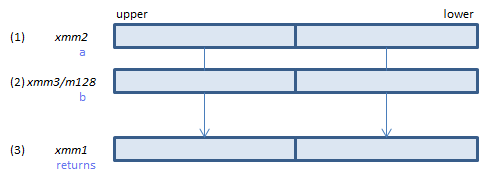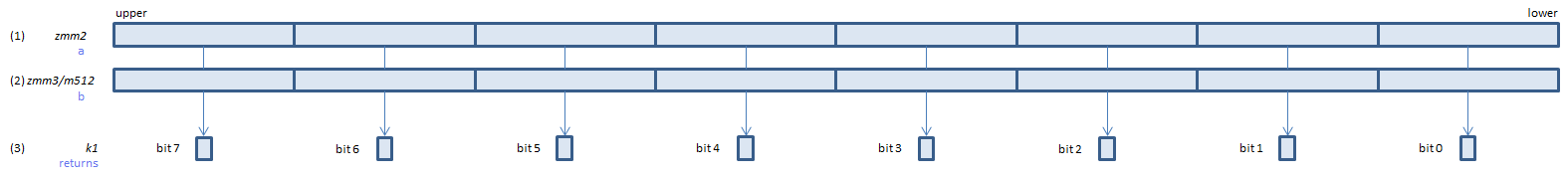﻿ vcmpngepd

## VCMPNGEPD - CoMPare Not Greater Equal Packed Double

VCMPNGEPD xmm1, xmm2, xmm3/m128    (V1
__m128d _mm_cmpnge_pd(__m128d a, __m128d b)    (S2For each double, if (1) < (2) or either or both of (1)(2) is NaN, set 1, else set 0, to all bits of the corresponding double of (3).
VCMPNGEPD ymm1, ymm2, ymm3/m256    (V1
__m256d _mm256_cmp_pd(__m256d a, __m256d b, _CMP_NGE_US)For each double, if (1) < (2) or either or both of (1)(2) is NaN, set 1, else set 0, to all bits of the corresponding double of (3).
VCMPNGEPD k1{k2}, xmm2, xmm3/m128/m64bcst    (V5+VLFor each double, if (1) < (2) or either or both of (1)(2) is NaN, set 1, else set 0, to the corresponding bit of (3).
If k2 bit is 0, the comparison is not done and the corresponding bit of (3) is set to zero. Upper bits of (3) are zero cleared.
VCMPNGEPD k1{k2}, ymm2, ymm3/m256/m64bcst    (V5+VLFor each double, if (1) < (2) or either or both of (1)(2) is NaN, set 1, else set 0, to the corresponding bit of (3).
If k2 bit is 0, the comparison is not done and the corresponding bit of (3) is set to zero. Upper bits of (3) are zero cleared.
VCMPNGEPD k1{k2}, zmm2, zmm3/m512/m64bcst{sae}    (V5